# Triangular Trinity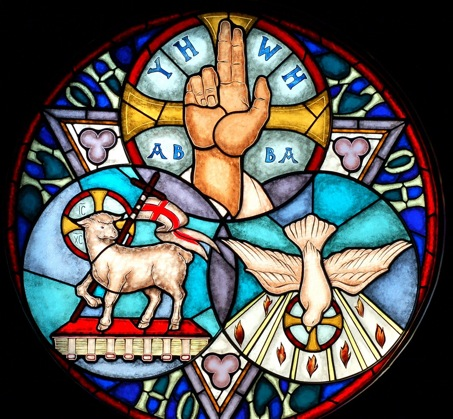Christians understand God to be a Trinity: the Father, the Son and the Holy Spirit. They are believed to be "co-equal, co-eternal and consubstantial". This pillar of Christian theology is given unusual support through English gematria. The universal visual symbol of the Trinity is the triangle, the very figure suggested by the numerical values of the English names we normally use for each of the three Persons.

The Names

The English names most often used for the three Persons of the Trinity are 'God', 'Jesus Christ' and 'the Holy Spirit'. 

First of all note that the names can describe triangle 6, with one word for each counter and each name completing one row.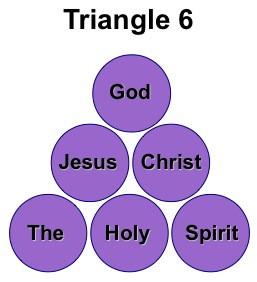This arrangement has each divine name in the order of its placing within the Trinity. So Jesus Christ is the second part of the Trinity and is placed in row two.

These names are also imprinted with a triangular watermark, only visible when they are combined to form a trinity.

The Numerical Values

Appropriately, three numeration schemes are involved: the standard, ordinal and reduced value systems, adapted to modern English from Hebrew and Greek, where they have been employed since biblical times.  Therefore the numeration schemes are long established and in fact predate the English language itself. 

When the three names are numerated under the three schemes, we obtain these numbers:

 Standard Value Ordinal Value Reduced Value God (s)                   =     71 God (o)                  =   26 God (r)                   = 17 Jesus Christ (s)      =   925 Jesus Christ (o)     = 151 Jesus Christ (s)      = 43 The Holy Spirit (s)  = 1489 The Holy Spirit (s)  = 184 The Holy Spirit (s)  = 76

Only one of these numbers is triangular. It is 1489, which can be displayed as a centred triangle. This is not particularly unexpected, as within this range of numbers about 1 in every 20 should be either a triangle or a centered triangle, so finding that one out of nine numbers is a triangle is fairly normal. 

However, when we combine the three numbers representing the three Persons of the Trinity - this combination reflecting the three-in-one nature of the Trinity - we find that three triangles suddenly appear. Here is the table again, showing the sum of the three numerical values for each name.

 Standard Value Ordinal Value Reduced Value God (s)                   =     71 God (o)                  =   26 God (r)                   = 17 Jesus Christ (s)      =   925 Jesus Christ (o)     = 151 Jesus Christ (s)      = 43 The Holy Spirit (s)  = 1489 The Holy Spirit (s)  = 184 The Holy Spirit (s)  = 76 Sum                       = 2485 Sum                       = 361 Sum                       = 136

Each sum is discussed below.

Standard Value

The sum of the three standard values is 2485, a numerical triangle, as shown below.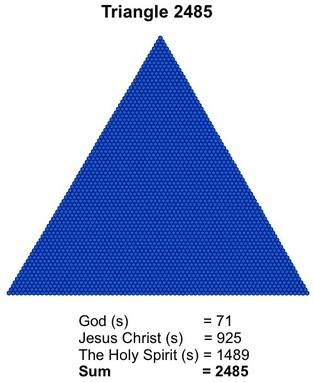An important class of triangular numbers is the 'G' triangles, which self-reflect to form the Star of David and which are the basis for snowflakes and trefoils. Only one in three triangles is a 'G' triangle and 2485, the 70th member of the sequence of triangular numbers, is one of them. The fact that 2485 is a 'G' triangle is of particular significance, because of the central role G-triangle geometry plays in the code.

Ordinal Value

The sum of the ordinal values is 361, which, as shown, is a centred triangle.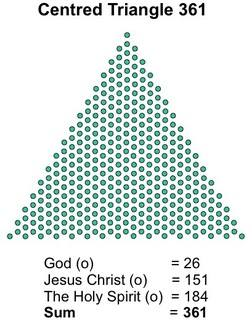The centred triangles, like stars, snowflakes and trefoils, are also related to 'G' triangles and can be created from them by subtraction of units. The 'G' triangle behind centred triangle 361 is triangle 1081. 

Reduced Value

The reduced values sum to 136, which is both a triangle and a centred triangle.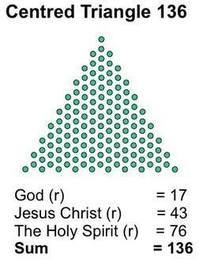It turns out that 136 is also a 'G' triangle and of course as a centred triangle it is associated with another 'G' triangle, triangle 406.

Three Quick Calculations

The triangle is the perfect symbol of the Trinity. What are the odds then that all three sums should be triangles? This is quite simple to calculate, using the binomial theorem.

Over the range 1 to 2485 there are 108 numbers that are either triangular, centred triangular or both, which means that only one out of 23 numbers randomly drawn from a bag containing the numbers 1 to 2485 should be triangular. For these calculations I will include the fourth system of numeration I use, for fairness.

a) The odds against three of the four systems giving totals summing to a triangular number are 1 in 3200 (for the three traditional systems only it is 1 in 12000).

b) The odds against three of the four systems giving totals summing to either a 'G' triangle or a centred triangle are 1 in 16500 (1 in 64500 for the three traditional systems).

c) The odds against two of the four systems producing a number that is a 'G' triangle are 1 in 1800 (1 in 3600 for the three traditional systems).

In other words it is by design. 

Summary

It is no accident that we find the three most popular English names for each Person of the Trinity summing to produce three triangles. As I show here, God has identified himself as the author of the New Bible Code, and the triangles He has woven into His name are not only a conscious reference to the Trinity - they also have the same pedigree as the stars, snowflakes and trefoils that decorate the rest of the New Bible Code.

Bill Downie 15/10/15

Notes

1. The wordings are from the NIV Bible, the vehicle for the new Bible Code. In the KJV Bible, the Holy Spirit was known as the Holy Ghost, a version of the name that has now fallen out of favour.

2. Those three Hebrew schemes were themselves adapted from schemes used by the Greeks.

3. This may lead some to argue that the spellings of these names were consciously manipulated over the years to give triangles. Notwithstanding the fact that the term 'Holy Ghost', which gives different numerical values, was widespread until recently, this scenario is highly improbable. I deal with the argument in more detail here.

4. Several of these numbers have other important geometric properties, as shown on this website.

5. 361 is also a square number, since it is 19 x 19.

6. Including the other names by which we call each Person of the Trinity in the calculations would greatly shorten these odds, but these are currently the most popular names by some margin and are likely to be for some time. As always the names or titles that are used most often today and that are found in the NIV Bible consistenly form the basis of the code. Indeed these are some of the features that identify it as a code.